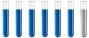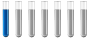## Question based on Titration and pH computation

Chemistry and homework help forum.

Organic Chemistry, Analytical Chemistry, Biochemistry, Physical Chemistry, Computational Chemistry, Theoretical Chemistry, High School Chemistry, Colledge Chemistry and University Chemistry Forum.

Share your chemistry ideas, discuss chemical problems, ask for help with scientific chemistry questions, inspire others by your chemistry vision!

Please feel free to start a scientific chemistry discussion here!

Discuss chemistry homework problems with experts!

Ask for help with chemical questions and help others with your chemistry knowledge!

Moderators: Xen, expert, ChenBeier

Dhamnekar Winod
Sr. Staff MemberPosts: 246
Joined: Sat Nov 21, 2020 10:14 am
Location: Mumbai[Bombay] and Mumbai Suburb,, Maharashtra State,India

### Question based on Titration and pH computation

Dissolve 2.0 g of the unknown monobasic acid sample in 100 ml of water. A 20 ml portion of this solution requires 15 ml of 0.12 M NaOH solution to reach the titrations equivalence point. If the molecular mass of the acid is 122 g/mol, determine the purity (%) of the acid.

How to answer this question? What is the answer to this question? I am working on this question. Any chemistry help, hint or even correct answer will be accepted.
Any science consists of the following process. 1) See 2)Hear 3)Smell,if needed 4)Taste, if needed 5)Think 6)Understand 7)Inference 8)Take decision [Believe or disbelieve, useful or useless, true or false, cause or effect, any other criteria]
ChenBeier
Distinguished MemberPosts: 1420
Joined: Wed Sep 27, 2017 7:25 am
Location: Berlin, Germany

### Re: Question based on Titration and pH computation

Compare the theoretical consumption with the real one.
Calculate the moles you used and the moles of the NaOH react.
Dhamnekar Winod
Sr. Staff MemberPosts: 246
Joined: Sat Nov 21, 2020 10:14 am
Location: Mumbai[Bombay] and Mumbai Suburb,, Maharashtra State,India

### Re: Question based on Titration and pH computation

Solution:

The titration calculations for NaOH:

For 20 ml acid solution: 15 ml 0.12 mol NaOH required

So, the number of base equivalents = 0.12 × 0.015 = 1.8 × 10⁻³ equivalent

So, in 20 ml of acidic solution 1.80 x 10⁻³ equivalent of acids

Therefore molarity of acid = 9 × 10⁻³ equivalent,

In a 2 g sample:

Acid mass = 9 × 10⁻³ × 122 = 1.098 g

% Purity = 1.098/2 × 100 = 54.9%
This is weak acid strong base titration.
Any science consists of the following process. 1) See 2)Hear 3)Smell,if needed 4)Taste, if needed 5)Think 6)Understand 7)Inference 8)Take decision [Believe or disbelieve, useful or useless, true or false, cause or effect, any other criteria]
ChenBeier
Distinguished MemberPosts: 1420
Joined: Wed Sep 27, 2017 7:25 am
Location: Berlin, Germany

### Re: Question based on Titration and pH computation

Correct.
althyrewarding
NewbiePosts: 1
Joined: Thu Feb 16, 2023 12:41 am
Location: United States
Contact:

### Re: Question based on Titration and pH computation

Determine the acid's purity (in percent) if its molecular mass is 122 g/mol. We need a strategy for responding to this inquiry. Do you know the solution to this puzzle? I'm trying to figure out the answer to it. We will gladly take any chemical advice, suggestion, or even right answer.
ChenBeier
Distinguished MemberPosts: 1420
Joined: Wed Sep 27, 2017 7:25 am
Location: Berlin, Germany

### Re: Question based on Titration and pH computation

rosydam
NewbiePosts: 1
Joined: Sun Sep 17, 2023 8:17 pm
Contact:

### Re: Question based on Titration and pH computation

Dhamnekar Winod wrote: Mon Aug 23, 2021 1:41 am Dissolve 2.0 g of the unknown monobasic acid sample in 100 ml of water. A 20 ml portion of this solution requires 15 ml of 0.12 M NaOH solution to reach the titrations equivalence point. If the molecular mass of the acid is 122 g/mol, determine the purity (%) of the acid.

How to answer this question? What is the answer to this question? I am working on this question. Any chemistry help, hint or even correct answer will be accepted.
To determine the purity of the unknown monobasic acid sample, you can follow these steps:

Calculate the number of moles of NaOH used: Since 20 mL of the unknown acid solution required 15 mL of 0.12 M NaOH to reach the titration equivalence point, you can calculate the moles of NaOH used:

Moles of NaOH = (0.12 moles/L) x (0.015 L) = 0.0018 moles

Determine the number of moles of acid: Since the balanced chemical equation for the reaction between the acid and NaOH is 1:1, the moles of the acid are also 0.0018 moles.

Calculate the mass of the acid: To find the mass of the acid, you can use the formula:

Mass (g) = Moles x Molecular Mass

Mass (g) = 0.0018 moles x 122 g/mol = 0.2196 g

Calculate the purity of the acid: To find the purity percentage, divide the mass of the pure acid by the mass of the sample (2.0 g) and multiply by 100:

Purity (%) = (0.2196 g / 2.0 g) x 100% ≈ 10.98%

So, the purity of the unknown monobasic acid sample is approximately 10.98%.
ChenBeier
Distinguished MemberPosts: 1420
Joined: Wed Sep 27, 2017 7:25 am
Location: Berlin, Germany

### Re: Question based on Titration and pH computation

No, you forget only a sample of 20 ml was titrated. The whole sample is 100 ml. So you have to multiply your result times 5. The solution was already given above.
Gomez22
NewbiePosts: 1
Joined: Tue Sep 19, 2023 4:19 am
Contact:

### Re: Question based on Titration and pH computation

To clarify, if the concentration of sulfuric acid in the 20 ml titrated portion was determined to be, for example, 0.1 M, then the concentration of sulfuric acid in the whole 100 ml sample would be 0.1 M multiplied by 5, resulting in a concentration of 0.5 M.
ChenBeier
Distinguished MemberPosts: 1420
Joined: Wed Sep 27, 2017 7:25 am
Location: Berlin, Germany

### Re: Question based on Titration and pH computation

No, the concentration in the 20 ml is 0,1 M = 0,1 mol/l , so in 100 ml its also 0,1 M. Concentration will not change if a small amount is taken out of a bigger volume. But it was original 2g unknown acid dissolved in 100 ml. 20 ml means 1/5 was titrated. To get the whole mass of the dissolved acid it has to be multiplied by 5.